# Ray Optics: Refraction and Lenses

## Refraction and Lenses: Problem Set

#### Problem 1:

Light travels through a vacuum at a speed of 2.998 x 108 m/s. Determine the speed of light in the following media:

a. water (n = 1.333)
b. crown glass (n = 1.52)
c. cubic zirconia (n = 2.16)
d. diamond (n = 2.419)

#### Problem 2:

Consider the three diagrams below. Measure the angle of incidence and of refraction (in degrees) for each of the three diagrams.#### Problem 3:

A ray of light travels through air (n = 1.00) and approaching the boundary with water (n = 1.33). The angle of incidence is 45.0°. Determine the angle of refraction.

#### Problem 4:

A light ray is passing through water (n=1.33) towards the boundary with a transparent solid at an angle of 56.4°. The light refracts into the solid at an angle of refraction of 42.1°. Determine the index of refraction of the unknown solid.

#### Problem 5:

During a physics lab, Ray Zuvlight observes a laser line passing through an unknown material towards a boundary with air with an angle of incidence of 24.5°. The light ray emerges into the air with an angle of refraction of 33.8°. Determine the index of refraction of the unknown material.

#### Problem 6:

Light in air approaches the boundary of oil at an angle of 36.1 degress with respect to the normal. The light travels at a speed of 2.27 x 108 m/s through the oil. Determine the angle of refraction.

#### Problem 7: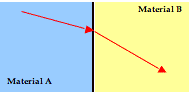The diagram at the right shows light refracting from material A into material B. The index of refraction of material A is 2.24. Use your protractor to measure angles and determine the index of refraction of material B. (HINT: The angle measures are multiples of 15 degrees.)

#### Problem 8: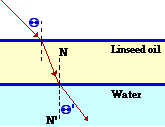The diagram at the right shows a ray of light traveling through air towards a thin layer of linseed oil (n = 1.50) resting on top of water (n=1.33). The light ray approaches the linseed oil at an angle of incidence of 48.2°.
a. Determine the angle of refraction at the air-linseed oil boundary.
b. Determine the angle of refraction at the linseed oil-water boundary.

#### Problem 9: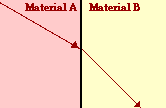A light ray is traveling through crown glass (n = 1.52) and approaching the boundary with water (n = 1.33) as shown in the diagram at the right.
a. Use a protractor to measure the angle of incidence of the light ray in the crown glass.
b. Calculate the angle of refraction of the light ray as it enters into the water.

#### Problem 10: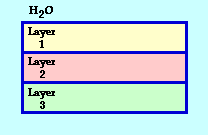The diagram at the right shows series of transparent materials that form layers on top of each other and are surrounded by water (n=1.33). Layer 1 has an index of refraction of 1.91; layer 2 has an index of refraction of 1.52; layer 3 has an index of refraction of 1.36. A light ray in water approaches the boundary with layer 1 at 62.8 degrees.
a. Determine the angles of refraction for the light as it enters into each layer
b. Determine the angle of incidence of the light after it passes through each layer and strikes the boundary with the next layer.
c. Determine the angle of refraction for the light as it refracts out of layer 3 into the water.

#### Problem 11:A tall cup is partially filled with water (n=1.33) to a height of 7.80 cm (Hwater). The diameter (D) of the cup is 14.64 cm. A student looks downward just over the left rim of the cup at an angle of 40.47 degrees with the water's surface (theta). At this angle, the refraction of light at the water's surface just barely allows her to see the bottom-right corner of the cup. A sketch (not drawn to scale) of the path of light is shown at the right. Determine the height of the cup (Hcup) in centimeters.

#### Problem 12:

Ray Zuvlite is playing with his underwater laser. He descends beneath the water surface in his backyard pool to a vertical depth of 7.09 feet and directs the laser beam at an angle towards the pool's edge. The beam emerges from water (n=1.33) into air at the pool's very edge and projects onto a pool house which is located 17.21 feet horizontally from the pool's edge. The dot on the pool house is observed to be located at a vertical height of 8.75 feet. Determine the distance which Ray is located horizontally out from the edge of the pool.

#### Problem 13:

Determine the critical angle of the following materials when surrounded by air:
a. teflon (n = 1.38)
b. Pyrex glass (n = 1.47)
c. Polycarbonate glass (n = 1.59)
d. Sapphire gemstone (n = 1.77)
e. Diamond (n = 2.42)

#### Problem 14:

Determine the critical angle of the following materials when surrounded by water (n = 1.33):
a. teflon (n = 1.38)
b. Pyrex glass (n = 1.47)
c. Polycarbonate glass (n = 1.59)
d. Sapphire gemstone (n = 1.77)
e. Diamond (n = 2.42)

#### Problem 15:

In a physics lab, Jerome and Gavin are assigned the task of determining the critical angle of solid, transparent prism using laser light. When surrounded by air, light is observed to undergo total internal reflection when the angle of incidence is as low as 38°. Calculate the index of refraction of the material which the prism is made of.

#### Problem 16:

During a demonstration of fiber optics, Mr. H uses an acrylic light pipe to demonstrate total internal reflection for light as it navigates its way through a curved medium, entering at one end and exitting at the other with very little loss of light along the way.
a. If the acrylic has an index of refraction of 1.48, then determine its critical angle when surrounded by air.
b. Determine the critical angle of the acrylic light pipe when surrounded by water (n=1.33).

#### Problem 17: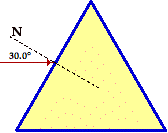Strontium titanate (SrTiO3) is a rare oxide mineral found naturally in tausonite crystals in Siberia. Because it has an index of refraction (n = 2.41) value similar to that of diamond, it is often used as a diamond substitute. Were it not for its greater physical density and its greater softness (it shows abrasions and scratches much more commonly under a microscope), a jeweler would have difficulty distinguishing between diamond and strontium titanate.

The triangular prism at the right is made of strontium titanate. A ray of light in air approaches the boundary at an angle of incidence of 30.0°. The ray strikes at the midpoint of one of the faces of the triangle.
a. Determine the angle of refraction upon entering into the prism.
b. Use geometric principles to determine the angle of incidence at the opposite side of the triangle.
c. Will the light ray refract out of the prism at this opposite face or will it undergo total internal reflection? Do the calculation and explain the answer.

#### Problem 18: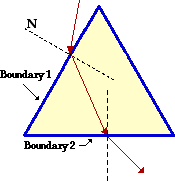In a physics lab, Anna Litical is studying the path of red laser light through a equiangular glass prism (n = 1.52). She observes that the light enters one face of the glass prism (boundary 1), refracts and then exits a second face of the glass prism (boundary 2). In her usual inquisitive manner, she ponders the question "I wonder if I could find an angle of approach to boundary 1 which would cause the light to undergo total internal reflection at boundary 2?" Calculate the angle of incidence at boundary 1 which would cause this total internal reflection at boundary 2.

#### Problem 19:

During a lens lab, Jerome and Michael placed a 4.5-cm tall night light bulb a distance of 42.8 cm from a lens. The image of the light bulb was inverted and appeared 26.5 cm from the lens.
a. Determine the focal length of the lens being by Jerome and Michael.
b. Determine the expected height of the image of the bulb.

#### Problem 20:

The converging lens on Julia's camera has a focal length of 52 mm. She uses the camera to take a picture of her friends at South's homecoming. Her friends are located a distance of 2.45 m from the camera as Julia focuses in on them. Calculate the distance from the lens to the film (i.e, the image distance).

#### Problem 21:

During an in-class exploration lab, students are looking through both converging and diverging lenses at various objects and observing the characteristics of the images. Moses becomes particularly intrigued by the diverging lens. He places it a distance of 4.8 cm from the lettering of his textbook and estimates that the image of the letters are one-fourth the size of the actual lettering.
a. Calculate the image distance which results when Moses holds the lens this close to the textbook.
b. Calculate the focal length of the lens.

#### Problem 22:

During the Finding Smiley Lab, Tyrone and Mia place a small light bulb at various distances from a converging lens. The light bulb has a smiley face marked on one of its side. Their goal is to locate the images of the light bulb by projecting the refracted light onto a note card.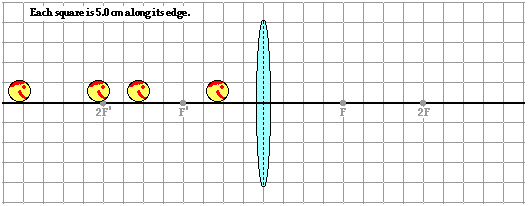The lens is known to have a focal length of 20. cm. Predict the image distances which Tyrone and Mia will likely determine when the smiley face is located a distance of …
a. … 60. cm from the lens.
b. … 40. cm from the lens.
c. … 30. cm from the lens.
d. … 10. cm fron the lens.

#### Problem 23:

The widest cinema screen in the world was reportedly constructed in New Zealand in 2007. The screen is 30.6 meters (100 feet) wide. Images from a 35-mm wide film are projected onto this screen. Suppose that the screen in the theater is located a distance of 46 m from the projector. Determine the magnification of the image and the focal length of the lens system.

#### Problem 24:

In an effort to read the miniaturized writing in Khaled's lab notebook, Mr. H pulls a magnifying glass from his drawer. He places the magnifying glass a distance of 6.3 cm from the paper and produces an upright image of the writing which is magnified by a factor of 4.8. Determine the focal length of the lens used by the magnifying glass.

#### Problem 25:

The focal point of a lens is located 17.8 cm away from its surface. The lens produces a virtual image which is 38.9 cm from the lens. Determine the object distance.

#### Problem 26:

A lens produces a virtual image located 33.8 cm from the lens when the object is located 18.5 cm from the lens. Determine the focal length of the lens. What kind of lens is it?

#### Problem 27:

Jill organizes a surprise 16th birthday party for her boyfriend Jack down at Bianchi's Pizza Parlor. In an effort to capture the nostalgic moment on film, Jill squeezes all of Jack's friends together on one side of the table. The 51.4 mm camera lens focuses the image of all objects onto a film negative which is 35.8 mm wide. If Jack and friends are located 4.62 m from the camera lens, to what maximum distance must they squeeze together in order to completely fit in the developed picture?

#### Problem 28:

In a converging lens lab, Anna Litical is challenged to determine the object distance which produces a real image which is magnified by a factor of five. The lens has a focal length of 12.0 cm. What object distance would you expect to produce this magnification?

#### Problem 29:

An upright image is reduced to one-fourth of the object’s height when the object is placed 26.9 cm from the lens. Determine the focal length of the lens.

#### Problem 30:

An upright image is magnified, appearing to be four times the size of the object’s when the object is placed 26.9 cm from the lens. Determine the focal length of the lens.

#### Problem 31:

Matthew's new Bug Habitat toy comes with a built in magnifier, allowing Matthew to get a close-up view of his captive ants, crickets, slugs, and other creatures. The magnifier has a focal length of 22 cm. Determine the distance which an ant must be located from the lens in order for it to appear magnified by a factor of 12.

#### Problem 32:

A lens produces an inverted image which is one-fifth the size of the object. The lens has a focal length of 22.8 cm. Determine the object distance which results in this magnification.